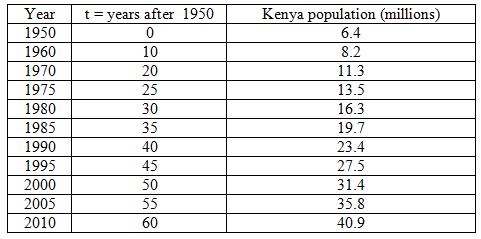## Exponential Relationships (3 of 6)

### Learning Objectives

• Use an exponential model (when appropriate) to describe the relationship between two quantitative variables. Interpret the model in context.

Let’s summarize what we have learned about exponential growth models:

The general form of an exponential growth model is y = C · bx.

• C is the initial value. It is the y-value when x = 0. It is also the y-intercept.
• b is the growth factor; it will always be greater than 1 in cases of growth. From the growth factor, we can determine the percentage increase in y for each additional 1 unit increase in x.

Let’s compare the general form of an exponential growth model to the general form for a linear model: y = a + bx.

• In the linear model, a is the initial value. It is the y-value when x = 0. It is also the y-intercept.
• b is the slope. From the slope, we can determine the amount and direction the y-value changes for each additional 1 unit increase in x.

Now we apply what we have learned about exponential growth to find a model for a set of data.

In this activity, you use a simulation to find an exponential model that fits the population growth of Kenya.

Here are the data graphed in the scatterplot in the simulation.Notice that the Kenyan population growth has a strong positive exponential form. Use the sliders in the simulation to adjust the values of C and b to find a reasonable exponential model that fits this data.